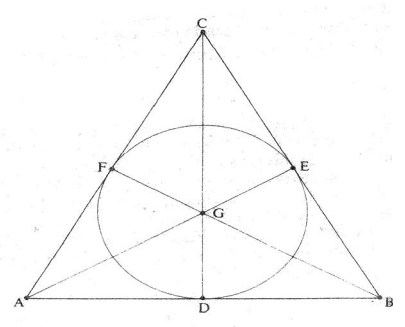Finite Geometry Notes   | Home | Site Map | Author |

## The Cube Model and Peano Arithmetic

### Note by Steven H. Cullinane on March 1, 2012

The eightfold cube  model of the Fano plane may or may not have influenced a new paper (with the date Feb. 10, 2011, in its URL) on an attempted consistency proof of Peano arithmetic—

The Consistency of Arithmetic, by Storrs McCall

"Is Peano arithmetic (PA) consistent?  This paper contains a proof that it is. ...

Axiomatic proofs we may categorize as 'syntactic', meaning that they concern only symbols and the derivation of one string of symbols from another, according to set rules.  'Semantic' proofs, on the other hand, differ from syntactic proofs in being based not only on symbols but on a non-symbolic, non-linguistic component, a domain of objects.    If the sole paradigm of 'proof ' in mathematics is 'axiomatic proof ', in which to prove a formula means to deduce it from axioms using specified rules of inference, then Gödel indeed appears to have had the last word on the question of PA-consistency.  But in addition to axiomatic proofs there is another kind of proof.   In this paper I give a proof of PA's consistency based on a formal semantics for PA.   To my knowledge, no semantic consistency proof of Peano arithmetic has yet been constructed.

The difference between 'semantic' and 'syntactic' theories is described by van Fraassen in his book The Scientific Image :

"The syntactic picture of a theory identifies it with a body of theorems, stated in one particular language chosen for the expression of that theory.  This should be contrasted with the alternative of presenting a theory in the first instance by identifying a class of structures as its models.  In this second, semantic, approach the language used to express the theory is neither basic nor unique; the same class of structures could well be described in radically different ways, each with its own limitations.  The models occupy centre stage." (1980, p. 44)

Van Fraassen gives the example on p. 42 of a consistency proof in formal geometry that is based on a non-linguistic model.  Suppose we wish to prove the consistency of the following geometric axioms:

A1.  For any two lines, there is at most one point that lies on both.
A2.  For any two points, there is exactly one line that lies on both.
A3.  On every line there lie at least two points.

The following diagram shows the axioms to be consistent:Figure 1

The consistency proof is not a 'syntactic' one, in which the consistency of A1-A3 is derived as a theorem of a deductive system, but is based on a non-linguistic structure.  It is a semantic as opposed to a syntactic proof.  The proof constructed in this paper, like van Fraassen's, is based on a non-linguistic component, not a diagram in this case but a physical domain of three-dimensional cube-shaped blocks. ....

... The semantics presented in this paper I call 'block semantics', for reasons that will become clear....  Block semantics is based on domains consisting of cube-shaped objects of the same size, e.g. children's wooden building blocks.  These can be arranged either in a linear array or in a rectangular array, i.e. either in a row with no space between the blocks, or in a rectangle composed of rows and columns.  A linear array can consist of a single block, and the order of individual blocks in a linear or rectangular array is irrelevant. Given three blocks A, B and C, the linear arrays ABC and BCA are indistinguishable.  Two linear arrays can be joined together or concatenated into a single linear array, and a rectangle can be re-arranged or transformed into a linear array by successive concatenation of its rows.  The result is called the 'linear transformation' of the rectangle.  An essential characteristic of block semantics is that every domain of every block model is finite.  In this respect it differs from Tarski’s semantics for first-order logic, which permits infinite domains.  But although every block model is finite, there is no upper limit to the number of such models, nor to the size of their domains.

It should be emphasized that block models are physical models, the elements of which can be physically manipulated.  Their manipulation differs in obvious and fundamental ways from the manipulation of symbols in formal axiomatic systems and in mathematics.  For example the transformations described above, in which two linear arrays are joined together to form one array, or a rectangle of blocks is re-assembled into a linear array, are physical transformations not symbolic transformations. ..."